#### How to Use Countif Function in Excel

In this lesson, you learn about the COUNTIF function in Excel.

A COUNTIF function counts the number of times within the specified range of cells that the specified value is performed as a criterion.

## Countif function syntax

=COUNTIF (range, criteria)

• Range – the range of cells that you want to count the data. There has to be an ongoing area.
• Criteria – a condition that must be met for cell counting. In the case of numeric values, the condition can look like e.g. 100.

## Countif function examples

Let’s analyze business examples of how to use countif function in Excel.

### Count string with exact string of text

You want to count cells where there is only REPORT text. The cells are in the C column.

The formula is =COUNTIF(C2:C10,”REPORT”)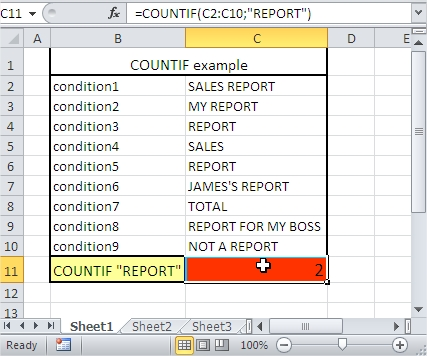### Greater than

You want to count cells whose value is greater than 10. The cells are in the C column.

The formula is =COUNTIF(C2:C10,”>”&10)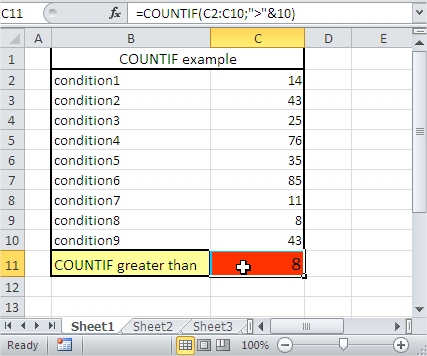### Greater than or equal to

You want to count cells whose values are greater than or equal to 10. The cells are in the C column.

The formula is =COUNTIF(C2:C10,”>=”&10)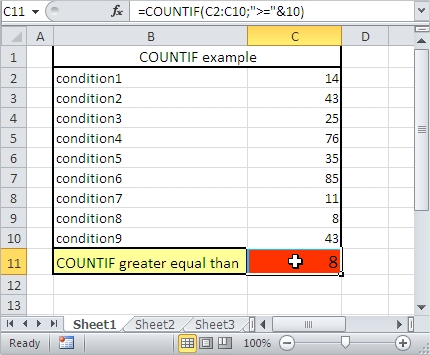### Not equal to

You want to count cells where the value is not equal to 10. The cells are in the C column.

The formula is =COUNTIF(C2:C10,”<>”&10)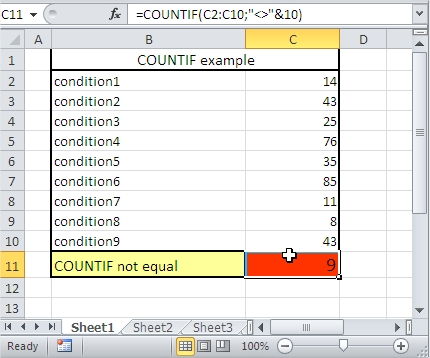### Less than x but greater than y

You want to count cells where value is less than 40 but more than 15. The cells are in the C column.

The formula is =COUNTIF(C2:C10,”<“&40)-COUNTIF(C2:C10,”<=”&15)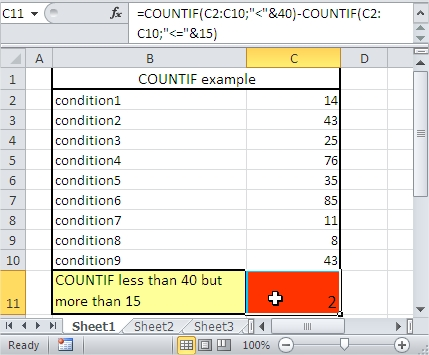### Countif plus countif

You want to count cells whose value is greater than 40 plus less than 15. The cells are in the C column.

The formula is =COUNTIF(C2:C10,”>”&40)+COUNTIF(C2:C10,”<“&15)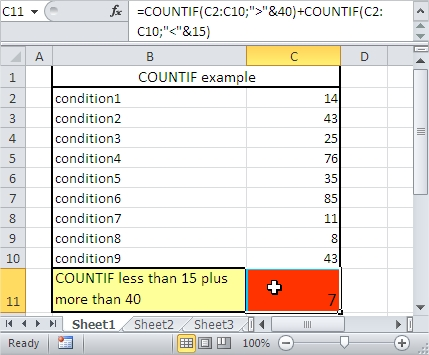### Count cells containing a string of text.

You want to count cells that contain REPORT text. The cells are in the C column. Use an asterisk in your formula. An asterisk replaces any other signs.

The formula is =COUNTIF(C2:C10,”*REPORT*”)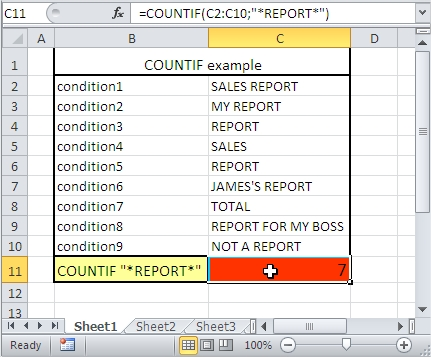### Count cells beginning with a string of text.

You want to count cells that begin to REPORT text. The cells are in the C column. Use an asterisk in your formula. An asterisk replaces any other signs.

The formula is =COUNTIF(C2:C10,”REPORT*”)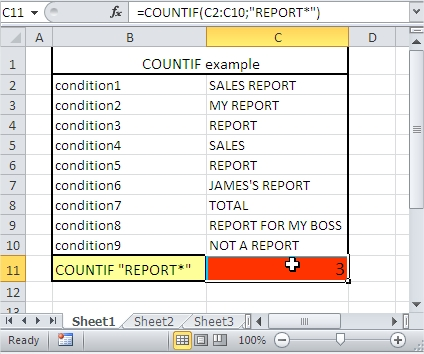### Count cells that end in a string of text.

You want to count cells that end in RT text. The cells are in the C column. Use an asterisk in your formula. An asterisk replaces any other signs.

The formula is =COUNTIF(C2:C10,”*RT”)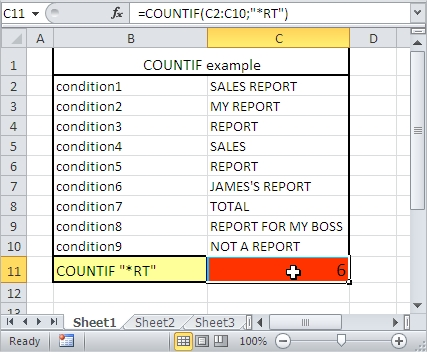### Count the cells that end in a string of text that contains 6 letters.

You want to count cells that end in RT text and contain 6 letters. The cells are in the C column. Use a question mark in your formula. A question mark replaces one sign.

The formula is =COUNTIF(C2:C10,”????RT”)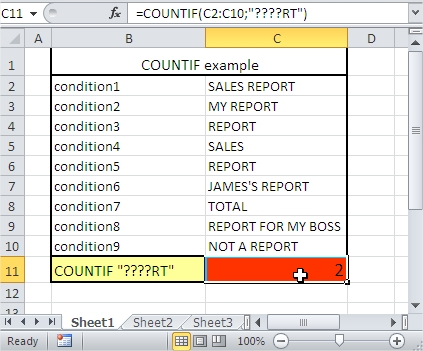### Count the cells containing any text.

You want to count cells that contain any text. The cells are in the C column. Use an asterisk in your formula. An asterisk replaces any other signs.

The formula is =COUNTIF(C2:C10,”*”)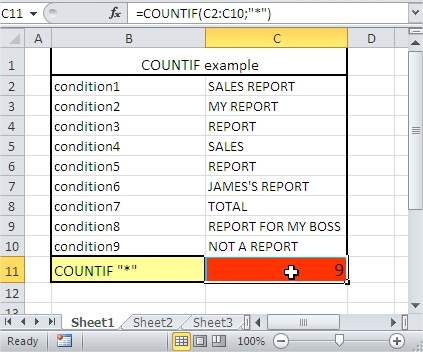### Count the cells that do not contain any text.

You want to count cells that do not contain any text. The cells are in the C column. Use an asterisk in your formula. An asterisk replaces any other signs. <> means not equal to.

The formula is =COUNTIF(C2:C10,”<>”&”*”)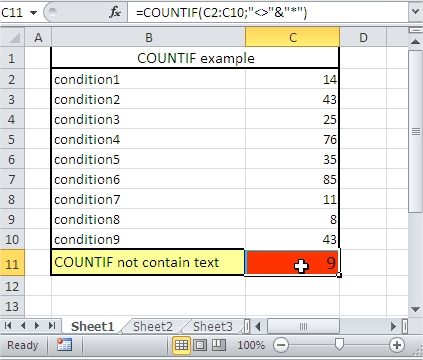### Greater than or equal to the value of the cell.

You want to count cells whose value is greater than or equal to the value of cell C12. The cells are in the C column.

The formula is =COUNTIF(C2:C10,”>=”&C12)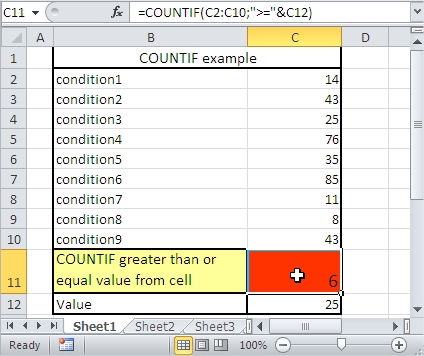### Greater than the value returned by the cell

You want to count cells whose value is greater than the value of the cell C12. The cells are in the C column.

The formula is =COUNTIF(C2:C10,”>”&C12)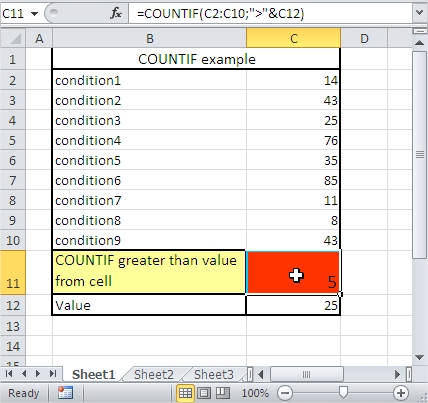Now you know how to use the COUNTIF function in Excel. You also know many COUNTIF formulas to use in your business cases.